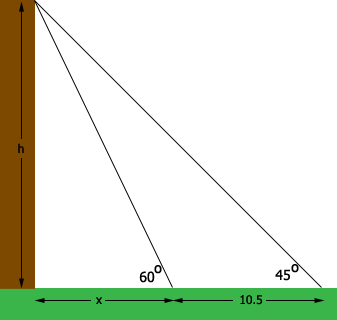SEARCH HOMEMath Central Quandaries & QueriesQuestion from Pandey: Hello sir plz solve this question. If the shadow of a tower is found to be 10.5m longer when the sun's altitude is 45°AND60°. Find the height of the tower.Hi,

In my diagram below the lengths re measured in meters, so the height of the tower is $x$ meters.From the diagram you can see that

$\tan\left( 60^2\right) = \frac{h}{x}.$

Write an expression for $\tan(45^{o}.$

Solve the two equations for $h.$

PennyMath Central is supported by the University of Regina and The Pacific Institute for the Mathematical Sciences.